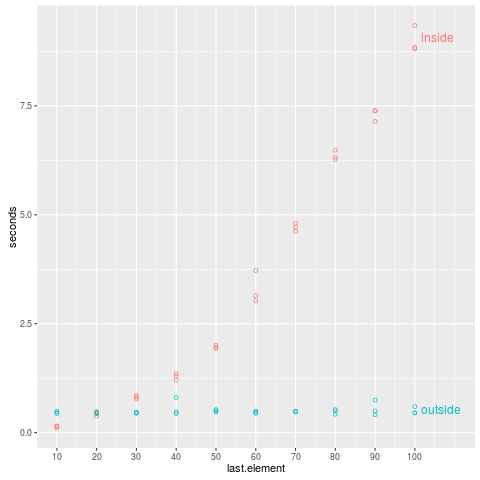Say you have a list of data tables, and you want to combine them. Of course, to combine data tables in R, we use rbind. But how?

I had two different students this week try the following, with rbind inside the for loop:

> system.time({
+   inside.dt <- NULL
+   for(i in 1:N){
+     inside.dt <- rbind(inside.dt, data.table(i, list.of.data[[i]]))
+   }
+ })
user  system elapsed
6.936   2.804   9.767
>


The code above is relatively slow because it is quadratic O(N^2) in the list size N. For each i from 1 to N, we must allocate a new data table of size O(i), and this is done N times. The quadratic time complexity comes from the fact that the size of the data table allocation gets bigger with each iteration of the for loop.

In contrast, consider the code below, which allocates a data table of the same (constant) size in each iteration of the for loop.

> system.time({
+   outside.dt.list <- list()
+   for(i in 1:N){
+     outside.dt.list[[i]] <- data.table(i, list.of.data[[i]])
+   }
+   outside.dt <- do.call(rbind, outside.dt.list)
+ })
user  system elapsed
0.38    0.06    0.44
>


I call this the “outside” version because the rbind is outside (after) the for loop. This timing is much faster, because it is linear time complexity. The combination step at the end do.call(rbind, outside.dt.list) is a linear time operation because it only needs to allocate one data table of the size of the result.

Both get the same result, as shown below.

> identical(inside.dt, outside.dt)
 TRUE
>


I used this R script to compute timings of these two algorithms for combining lists of various sizes (10 data.tables, 20, …, 100). The timings are plotted in the figure below, which clearly shows the quadratic time complexity of the inside version:Conclusion: use the version with rbind outside the for loop: do.call(rbind, list.of.data.tables) is fast because it is a linear time operation!